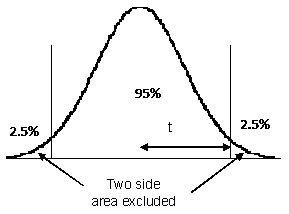Content DisclaimerCopyright @2020.All Rights Reserved.
StatsToDo : Probability of Student's t
Explanations, Calculations, Codes and Tables
 Introduction Calculations Codes Tables William Gosset was a brewer, but had an interest in statistics. He found the estimation of the probability for the standard deviate z unreliable if the observations were few. He derived a correction of the probability estimate according to sample size and called it t.  Gosset published his papers under the pseudonym of Student and this became known as Student's t. Student's t allows the use of a small number of measurements to estimate what may be true of the whole population.  This forms the basis of modern inferential statistics, where a small number of observations are made, and the results are generalized to the wider population.The t distribution curve is wider than the normal one.  Therefore, a larger area (or higher probability) of being greater than a particular deviate is obtained compared to the normal distribution. This difference varies with sample size (degrees of freedom), such that the probability of t approaches that of z when the sample size increases towards infinity. Conceptually, this is represented by the diagram to the left. With infinite degrees of freedom (i.e., a large sample size), the one tailed t and z have the same value for a particular probability, but with fewer cases, t will be larger than z in obtaining the same probability.    One and two tails When calculating t, a one tail or two tail model needs to be specify. A one tailed t is conceptually similar to the z, and assumes all the excluded values are on one side (tail) of the normal distribution, as shown in the following diagram.A two tailed t however, assumes the area excluded are on both sides (tails) of the t distribution, so that each side contains only half of the excluded area, as shown in the following diagram. In calculations involving the confidence interval, the two tailed t is usually used.   For examples : For a two tail model calculating 95% confidence interval using a sample of 21 cases, the t value for p=0.05 (p=0.025 excluded on each of the two tails) and degrees of freedom=20 is 2.09 is obtained. In other words, the 95% confidence interval is mean±2.09SD. For a one tail model, p=0.05 (all 5% excluded in one tail) the t value is 1.72. The 95% confidence interval is either -∞ to mean+1.72SD, or mean-1.72SD to +∞, depending on which tail is used. The other panels on this page are Calculations: Javascript program to calculate the probability of t Codes: R and Python codes to calculate the probability of t Tables: Tables for probability of t References https://en.wikipedia.org/wiki/Student%27s_t-distributionWikipedia on t Javascript algorithm adapted from Press WH, Flannery BP, Teukolsky SA, and Vetterling WT. (1994) Numerical recipes in Pascal. Cambridge University Press ISBN 0-521-37516-9. p.189